# Homework questions Homework questions Homework questions 2 5

• Slides: 26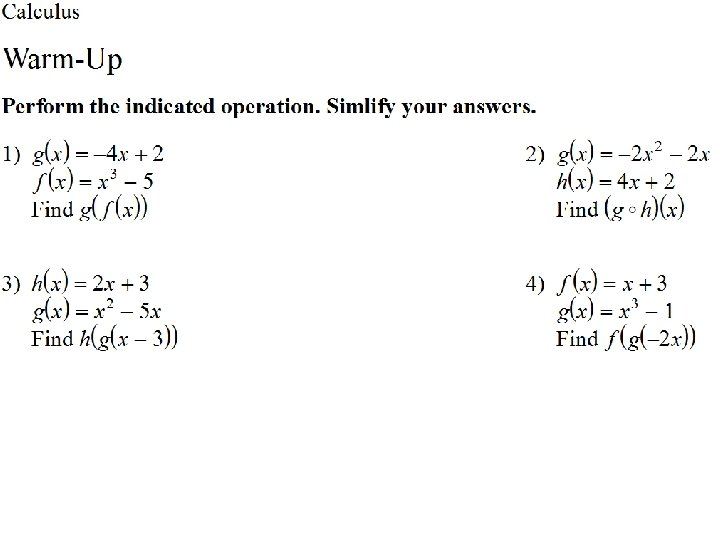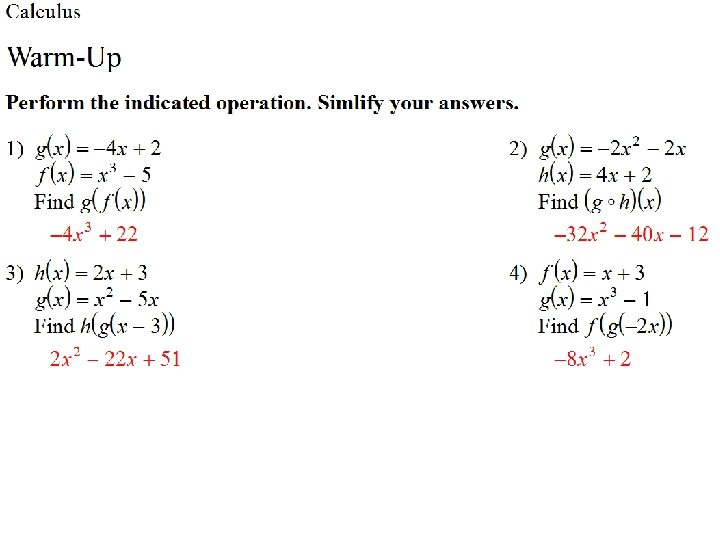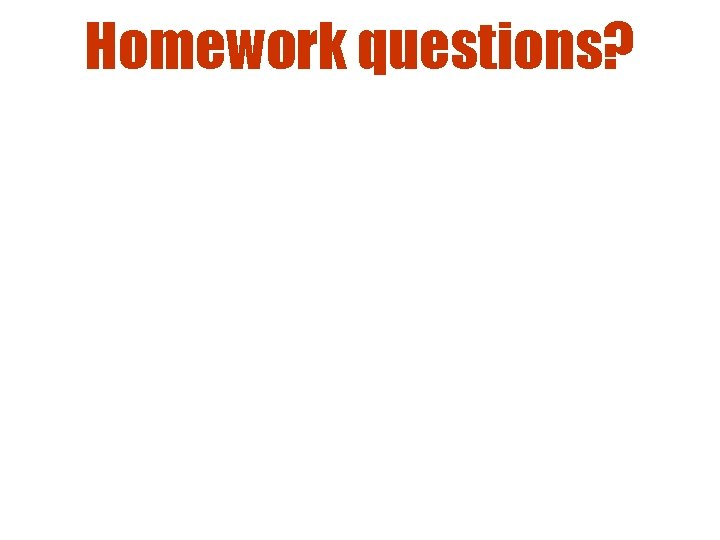Homework questions?Homework questions?Homework questions?2 -5: Implicit Differentiation Objectives: • Define implicit and explicit functions • Learn implicit differentiation © 2002 Roy L. Gover (www. mrgover. com)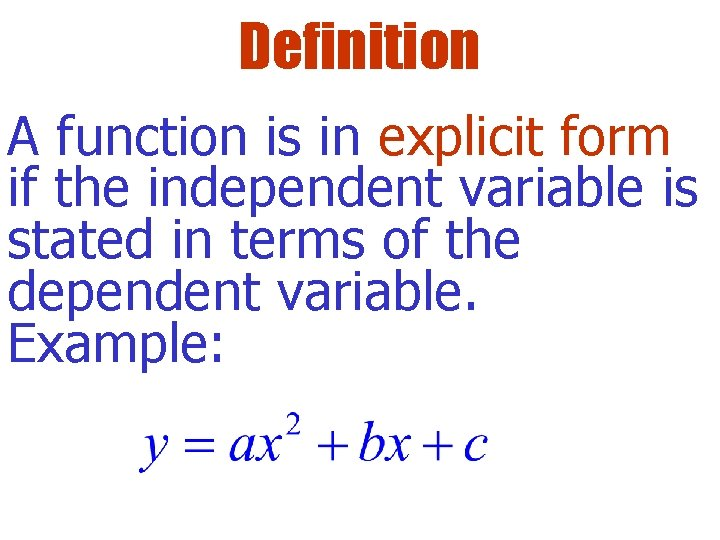Definition A function is in explicit form if the independent variable is stated in terms of the dependent variable. Example: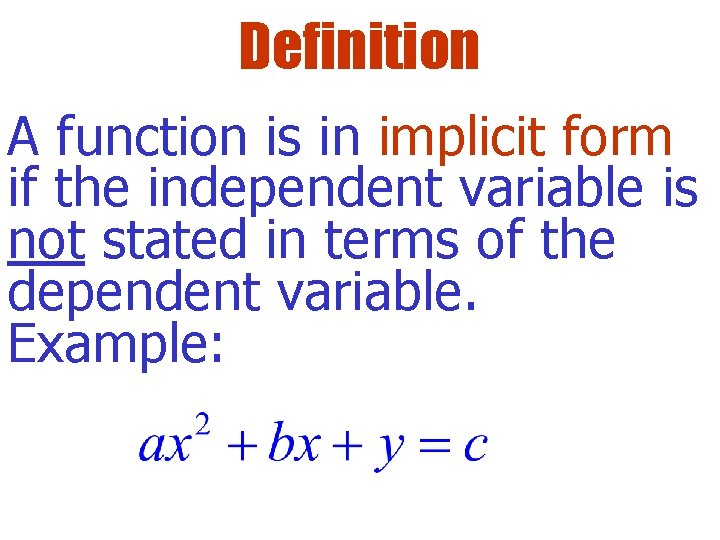Definition A function is in implicit form if the independent variable is not stated in terms of the dependent variable. Example: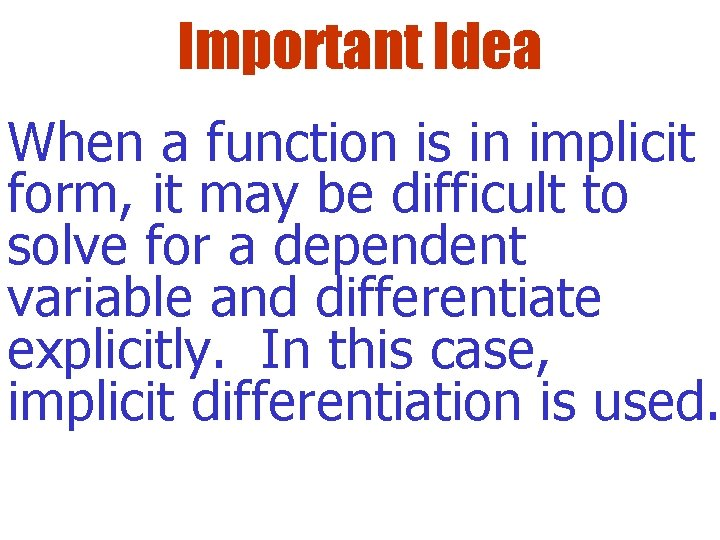Important Idea When a function is in implicit form, it may be difficult to solve for a dependent variable and differentiate explicitly. In this case, implicit differentiation is used.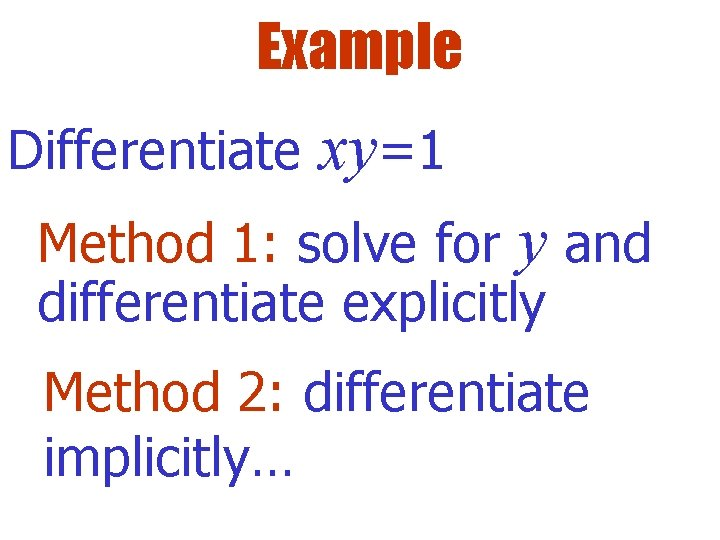Example Differentiate xy=1 Method 1: solve for y and differentiate explicitly Method 2: differentiate implicitly…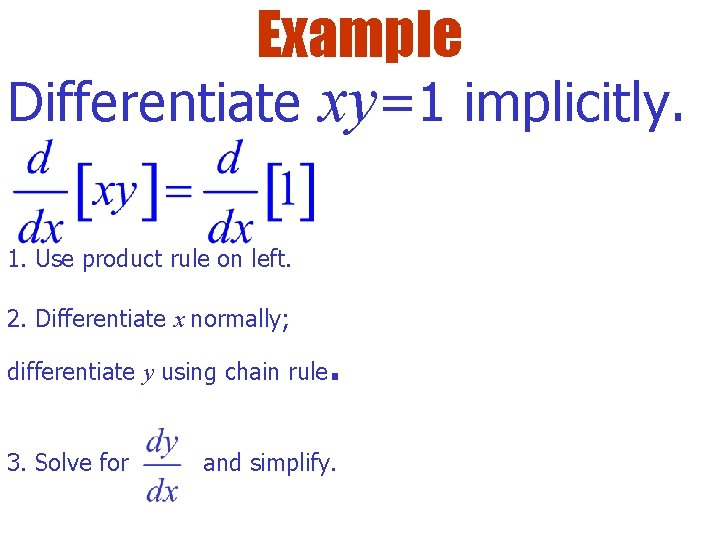Example Differentiate xy=1 implicitly. 1. Use product rule on left. 2. Differentiate x normally; differentiate y using chain rule 3. Solve for . and simplify.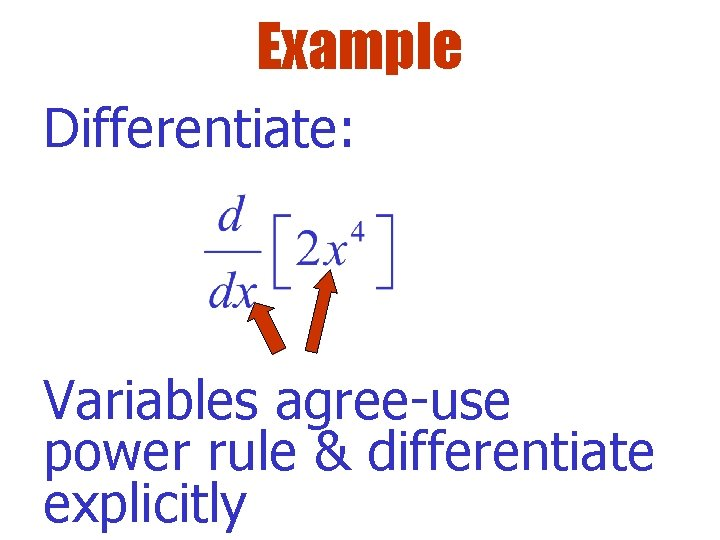Example Differentiate: Variables agree-use power rule & differentiate explicitlyExample Differentiate: Variables disagree-use power rule & differentiate implicitly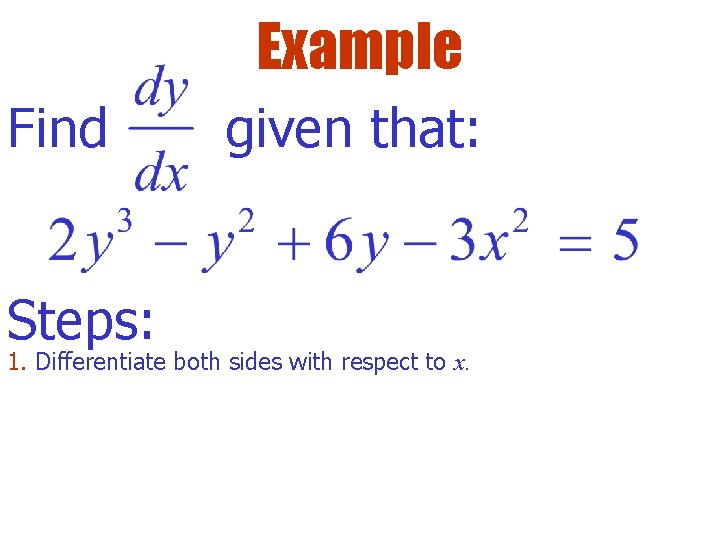Example Find Steps: given that: 1. Differentiate both sides with respect to x.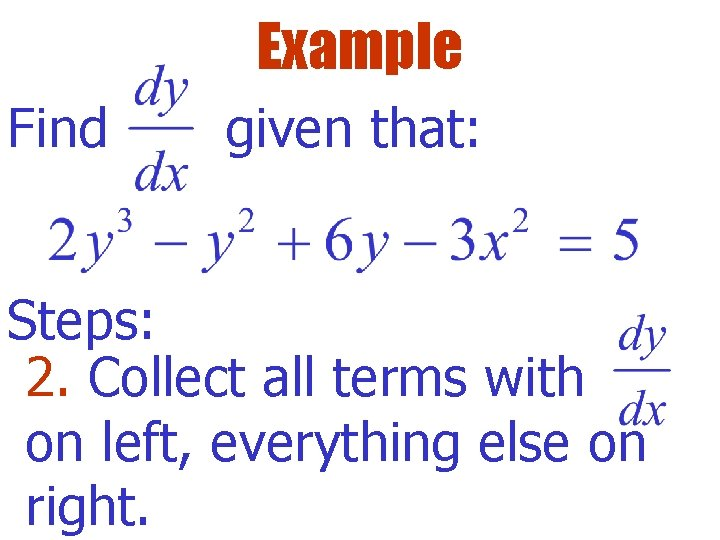Example Find given that: Steps: 2. Collect all terms with on left, everything else on right.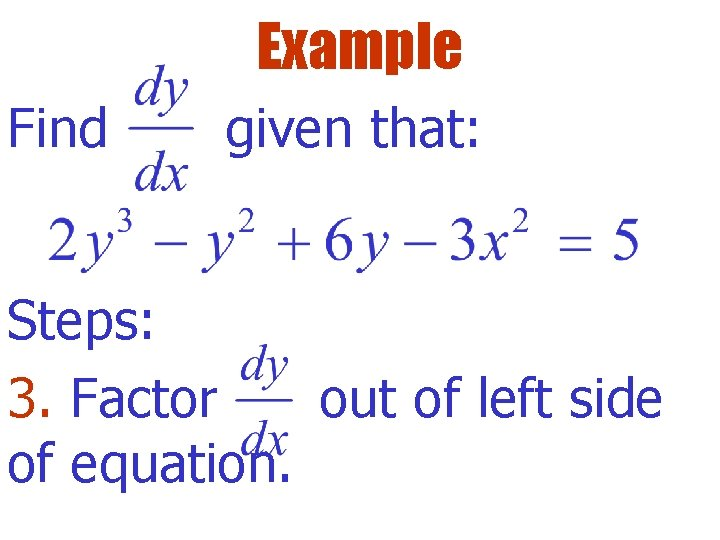Example Find given that: Steps: 3. Factor out of left side of equation.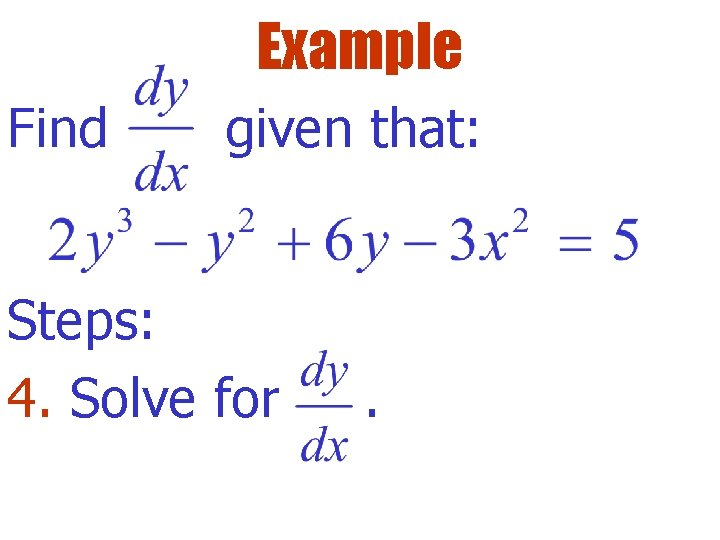Example Find given that: Steps: 4. Solve for .Try This Find : Hint: differentiate implicitly using the product rule.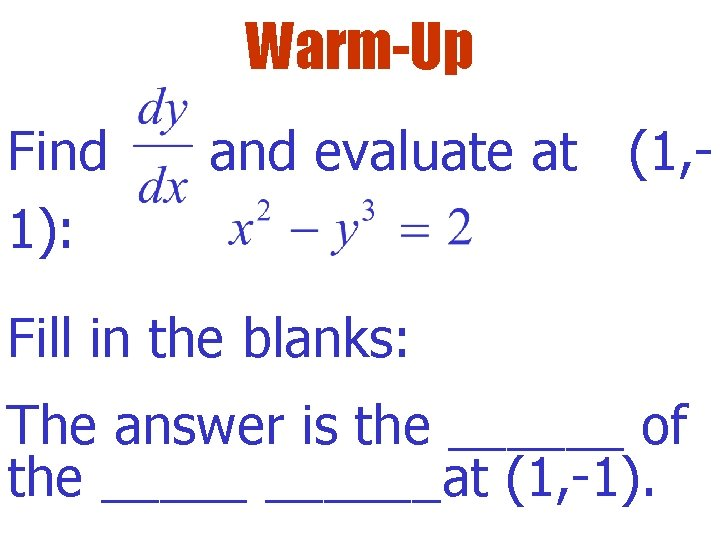Warm-Up Find 1): and evaluate at (1, - Fill in the blanks: The answer is the ______ of the ______at (1, -1).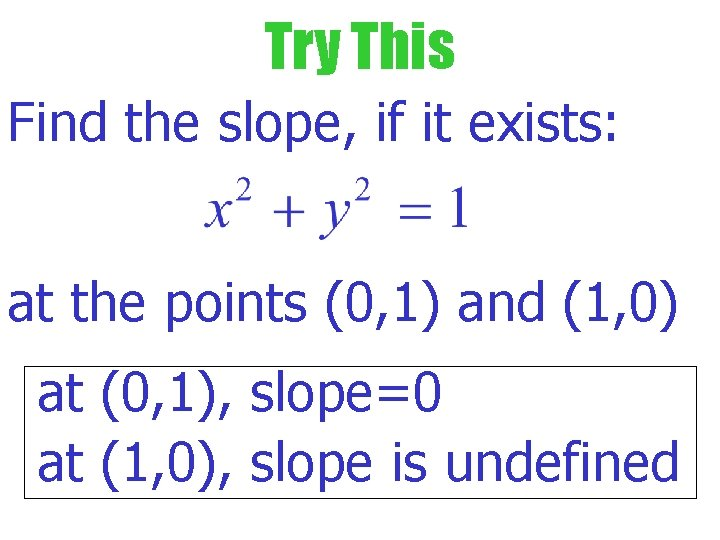Try This Find the slope, if it exists: at the points (0, 1) and (1, 0) at (0, 1), slope=0 at (1, 0), slope is undefinedTry This Find the equation of the line tangent to the graph: at the pointTry This Find implicitly:Lesson Close When would you use implicit differentiation to find the derivative?Assignment 1. 146/1 -15 odd, 35, 37, 41